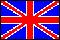ISSN 2079-3537

Scientific Visualization
 Issue Year: 2016 Quarter: 2 Volume: 8 Number: 2 Pages: 37 - 52
 Article Name: VISUALIZATION OF STABILITY AND CALCULATION OF THE SHAPE OF THE EQUILIBRIUM CAPILLARY SURFACE Authors: A.A. Klyachin (Russian Federation), V.A. Klyachin (Russian Federation), E.G. Grigorieva (Russian Federation) Address: A.A. Klyachin klyachin-aa@yandex.ru Volgograd State University, Volgograd, Russian Federation V.A. Klyachin klchnv@mail.ru Volgograd State University, Volgograd, Russian Federation E.G. Grigorieva e_grigoreva@mail.ru Volgograd State University, Volgograd, Russian Federation Abstract: The article is devoted to the 3D modeling of equilibrium capillary surfaces and visualize various effects associated with the stable or unstable. The construction of such a surface is reduced to finding solutions of some nonlinear partial differential equations satisfying certain boundary condition (on the border is defined by the contact angle between the liquid and the wetted solid wall of the capillary tube). The existence and uniqueness of the solution of this problem in sufficient detail are reflected, for example, in Robert Finn "Equilibrium capillary surfaces. Mathematical Theory" (see., Also references therein). To find approximate solutions we use the variational finite element method, in which the basis functions are chosen piecewise linear functions defined on a triangulation of a calculation domain. This approach was used in the calculations of the surface shape of minimal area. We obtained the following results For the above method of calculation we developed several program procedures to get calculation results in a convenient form for subsequent processing. The test calculations for some forms of sections of capillary tubes: hexagon, octagon, a square. We modeled unstable behavior of the capillary surface at the boundary point with a sufficiently small angle between the faces of the capillary tube and the critical value of the angle of contact. Approximately also calculated the height of raising liquid which was quite close to the value that is obtained by calculating the known physical formulas. It is, in a sense, confirms the correctness of the used calculation method. The formulas for the derivatives of functional area type of piecewise linear approximations of surfaces of arbitrary topology are obtained. These formulas are needed for the software implementation process iterations gradient descent. Implemented a system of classes for constructing approximate solutions of variational problems such as capillary surfaces. In addition, this class system allows numerical study of the stability of the extreme types of functional surfaces for a functional area type. Language: English